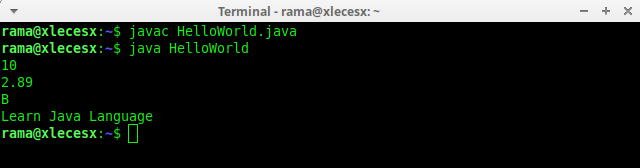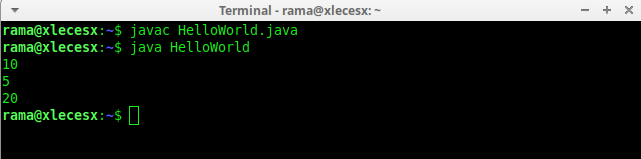# How to Declare Java Language VariablesVariables are identities used to hold a value. Technically, the variable refers to an address in the computer's memory. When we create a variable, a memory slot will be prepared to hold that value. Each variable has a name as the identity of that variable.

The contents of variables can change throughout the program code. For example, if I make the program calculate the area of a triangle, then I will prepare a variable length and width with the contents of numbers 10 and 12. Then, later the contents of the variable length and width can be changed by the numbers 20, 50, or other values.

Variables can also be used to hold input values, for example, we want the length and width to be filled by the user. Regarding how to input data into the Java program code, we will discuss it in a separate tutorial.

Before I continue, for those who are new to learning java, you should first read:

How To Install Java JDK and JRE

How To Add Java Jdk To Path Tutorial

How To Run Java Program Code (Compile Process)

### Rules for Naming Variables in Java

The variable naming rules refer to the identifier requirements that we discussed in the previous tutorial Understanding The Basics Structure And Java Syntax.

However, let me tell you again the rules for naming variables in the Java programming language:
• Variables can consist of letters, numbers and underscore characters (_).
• The first character of a variable can only be letters and underscore (_), cannot be numbers.
• Variables must be other than keywords. For example, we cannot use the word int as a variable name, because int is a keyword for integer data types.
• Variable names should be written using the camelCase writing style, where each word also starts with an uppercase letter, except the first word and no spaces. For example: car, carName, or sumDiscount.
When writing variables, there are 2 steps in almost all programming languages: declaration and initialization.

Declaration is the process of telling a Java compiler that we will create a variable. Here, java is a programming language with the concept of strongly typed programming language, which means that for each variable, the data type must be declared. Is it integer, float number, char, or something else.

The most commonly types of variables in java with examples
Data Type Size Description
byte 1 byte Stores whole numbers from -128 to 127
short 2 bytes Stores whole numbers from -32,768 to 32,767
int 4 bytes Stores whole numbers from -2,147,483,648 to 2,147,483,647
long 8 bytes Stores whole numbers from -9,223,372,036,854,775,808 to 9,223,372,036,854,775,807
float 4 bytes Stores fractional numbers. Sufficient for storing 6 to 7 decimal digits
double 8 bytes Stores fractional numbers. Sufficient for storing 15 decimal digits
boolean 1 bit Stores true or false values
char 2 bytes Stores a single character/letter or ASCII values

For example, in the following program code I declare 4 variables:
``````
class HelloWorld {
public static void main(String args[]){
int result;
double area;
char alphabet;
String activity;
}
}
``````

Here, int result will create a variable with int data type. This means that the result variable can only be filled with an integer. Area is a double type, so it can hold fractional numbers. Alphabet is type char, so it can hold 1 character. And activity has a String data type to be filled in with 1 sentence.

After a variable is declared, we can input or give the initial value into the variable. This initial assignment process is known as initialization.

The value provided must match the data type. Following is an example of the declaration and initialization process from the previous code:
``````
class HelloWorld {
public static void main(String args[]){
int result;
double area;
char alphabet;
String activity;

result = 10;
area = 2.89;
alphabet = 'B';
activity = "Learn Java Language";
}
}
``````

Now, each variable already contains a value. The equal sign (=) is an assignment operator to fill in a value. The assignment process is carried out from right to left. Result = 10 means that we assign the number 10 to the result variable.

To display the contents of a variable, we can use the System.out.println() command:
``````
class HelloWorld {
public static void main(String args[]){
int result;
double area;
char alphabet;
String activity;

result = 10;
area = 2.89;
alphabet = 'B';
activity = "Learn Java Language";

System.out.println(result);
System.out.println(area);
System.out.println(alphabet);
System.out.println(activity);
}
}
``````Variable declaration and initialization process can also be done at once in one line statement:
``````
class HelloWorld {
public static void main(String args[]){

int result = 10;
double area = 2.89;
char alphabet = 'B';
String activity = "Learn Java Language";

System.out.println(result);
System.out.println(area);
System.out.println(alphabet);
System.out.println(activity);
}
}
``````

We can also declare several variables in one command line, as long as those variables have the same data type. Here is an example:
``````
class HelloWorld {
public static void main(String args[]){
int a, b, c;

a = 22;
b = 9;
c = 86;

System.out.println(a);
System.out.println(b);
System.out.println(c);
}
}
``````

Once a variable is declared and given initial values, we also can change its value throughout the program code runs:
``````
class HelloWorld {
public static void main(String args[]){

int a, b, c;

a = 5;
b = a;
a = a + b;
c = b + b + a;

System.out.println(a);
System.out.println(b);
System.out.println(c);
}
}
``````

If we try to run the piece of java code above, then the result:In this tutorial, we have learned the meaning of variables and ways of writing a variable in the Java programming language.